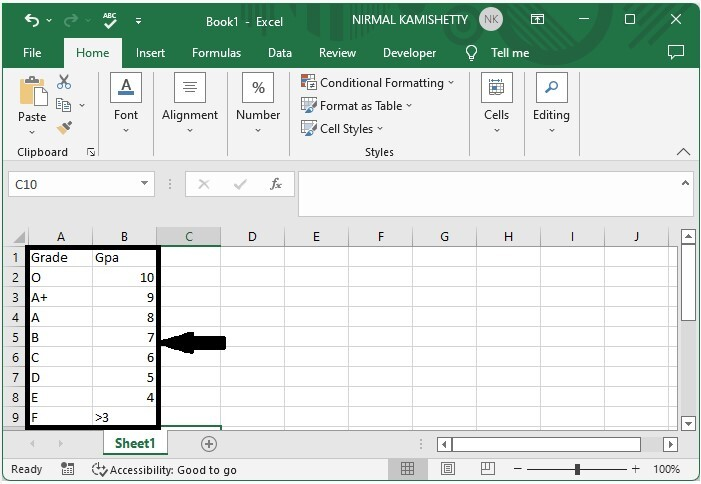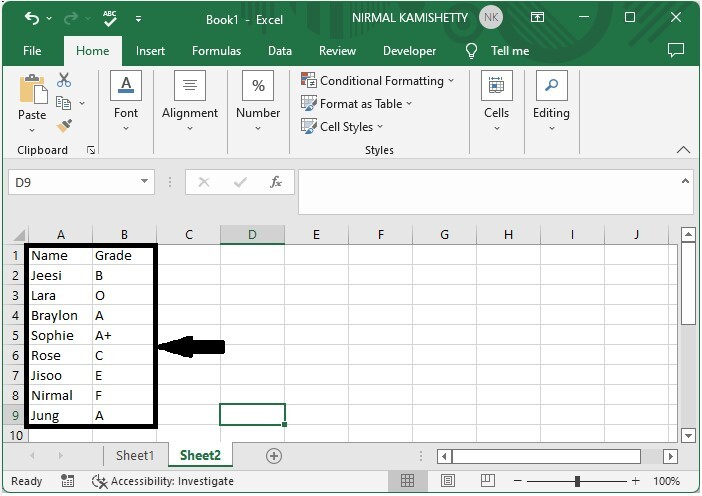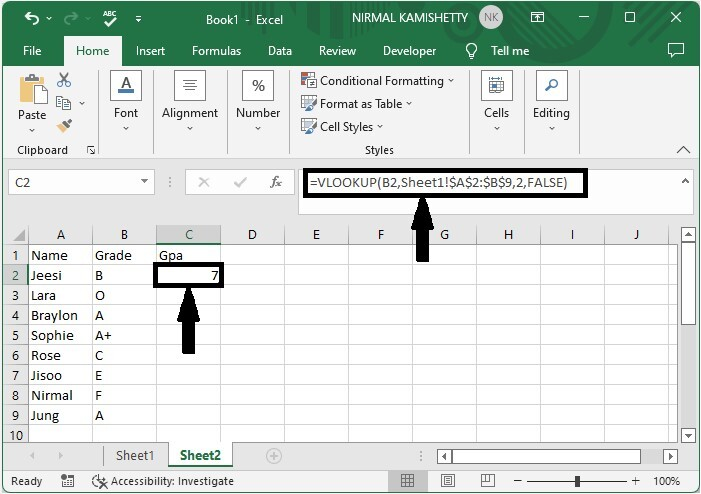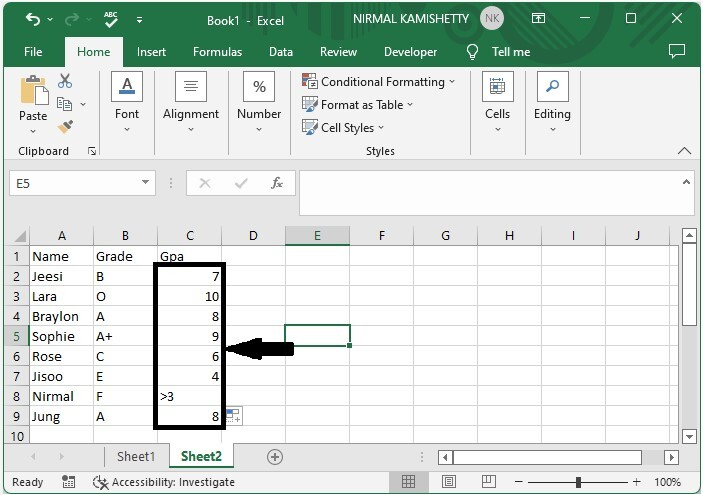# How to Convert Letter Grade to Number in Excel?

In general, we try to grade the test using a letter such as A+, A, B, and many others, and the minimum GPA is always linked to the grade. You may want to display them alongside the letter grade at times. Read this tutorial to learn how you can convert letter grades to numbers in Excel.

If we try to do this conversion in a manual way, then it can be time-consuming and sometimes inaccurate. So, we can use the VLOOKUP function to make our process faster and more accurate. Use VLOOKUP when you need to find things in a table or a range by row. For example, look up the price of an automotive part by the part number or find an employee's name based on their employee ID.

## Converting Letter Grade to Number in Excel

In this section, we will first make a list of grades and their corresponding numbers in the sheet. Then use the VLOOKUP formula to get the results. Let's go over a simple procedure for converting letter grades to numbers in Excel using the VLOOKUP function.

### Step 1

First, we need a table where the minimum GPA is connected to the letter grade, similar to the below image.Consider the following image of an excel sheet containing a list of letter grades.Now, in our case, cell C2, click on an empty cell and enter the formula as follows

=VLOOKUP(B2,Sheet1!$A$2:$B$9,2,FALSE) and click Enter to get our first result. In the formula, B2 is the address of the letter grade, Sheet1 is the sheet name of the grade table, and A2:B9 is the range of values in Sheet1.

Empty cell > Formula > Enter### Step 2

Then drag down from the first result to fill all the values, and our result will be similar to below image.## Conclusion

In this tutorial, we used a simple example to demonstrate how you can convert letter grades to numbers in Excel.

Updated on: 24-Feb-2023

916 Views